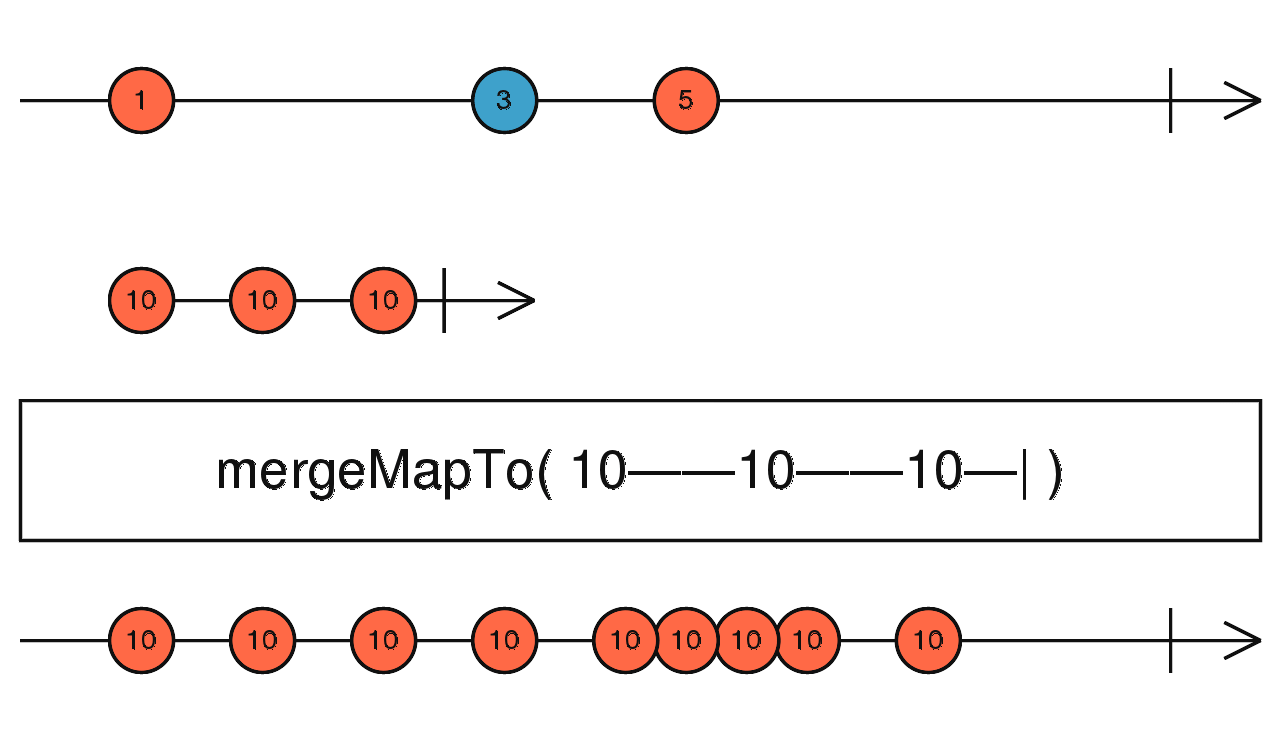/RxJS

# mergeMapTo

`function` `stable`

Projects each source value to the same Observable which is merged multiple times in the output Observable.

### `mergeMapTo<T, R, O extends ObservableInput<any>>(innerObservable: O, resultSelector?: ((outerValue: T, innerValue: ObservedValueOf<O>, outerIndex: number, innerIndex: number) => R) | number, concurrent: number = Number.POSITIVE_INFINITY): OperatorFunction<T, ObservedValueOf<O> | R>`

#### Parameters

 innerObservable An Observable to replace each value from the source Observable. resultSelector Optional. Default is `undefined`. Type: `((outerValue: T, innerValue: ObservedValueOf, outerIndex: number, innerIndex: number) => R) | number`. concurrent Optional. Default is `Number.POSITIVE_INFINITY`. Maximum number of input Observables being subscribed to concurrently.

#### Returns

`OperatorFunction<T, ObservedValueOf<O> | R>`: An Observable that emits items from the given `innerObservable`

## Description

It's like `mergeMap`, but maps each value always to the same inner Observable.Maps each source value to the given Observable `innerObservable` regardless of the source value, and then merges those resulting Observables into one single Observable, which is the output Observable.

## Example

For each click event, start an interval Observable ticking every 1 second

```import { fromEvent, interval } from 'rxjs';
import { mergeMapTo } from 'rxjs/operators';

const clicks = fromEvent(document, 'click');
const result = clicks.pipe(mergeMapTo(interval(1000)));
result.subscribe(x => console.log(x));```